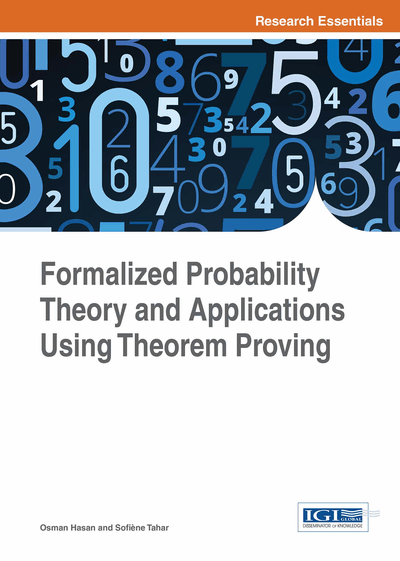# Probabilistic Analysis Using Theorem Proving

DOI: 10.4018/978-1-4666-8315-0.ch003
Available
\$37.50
No Current Special Offers

## Abstract

In this chapter, the authors first provide the overall methodology for the theorem proving formal probabilistic analysis followed by a brief introduction to the HOL4 theorem prover. The main focus of this book is to provide a comprehensive framework for formal probabilistic analysis as an alternative to less accurate techniques like simulation and paper-and-pencil methods and to other less scalable techniques like probabilistic model checking. For this purpose, the HOL4 theorem prover, which is a widely used higher-order-logic theorem prover, is used. The main reasons for this choice include the availability of foundational probabilistic analysis formalizations in HOL4 along with a very comprehensive support for real and set theoretic reasoning.
Chapter Preview
Top

## 3.1 Methodology

The methodology followed by this book is depicted in Figure 1. The rectangle with the dashed border in this figure represents the foundational requirements of conducting probabilistic analysis in a theorem prover. Like all system analysis tools, the input to this framework, represented by the oval blocks, is a description about the given system that needs to be analyzed and a set of properties that are required to be checked for the given system. These foundational theories provide the necessary tools to formally or mathematically express a system description and its desired properties in a format that is understood by the theorem prover. The formally expressed properties are then formally verified to hold for the given model within the sound environment. These proofs are the final outcome of the analysis and are given in the form of a proof script written in the language of HOL.

The foremost requirements, which are enclosed in the rectangle with dashed borders, are the theories of probability, Markov chains, information and reliability. However, all of these theories heavily rely upon the measure and Lebesgue integration theories to formalize the random variables, statistical properties and information measures. The Lebesgue integration theory also makes use of the measure theory as the integrals are defined for measurable functions with respect to measure functions. Finally, the measure theory, and more specifically the formalization of the Borel sigma algebra, requires various concepts from the topology theory, like the definition of open sets, and important properties from the theory of rational numbers, like the countability of the set of rational numbers and its density in the set of real numbers.

The formalization of the set of extended-real numbers, i.e, the set of real numbers augmented by the negative and positive infinity, is used as a basis for the development of the various theories of the framework to facilitate the verification of several properties, mainly convergence theorems, that would not have been possible to prove using the normal (finite) real numbers. This choice also aids the verification of the important Radon Nikodym theorem and definition of the Radon Nikodym derivative, which is a necessity to define most commonly used measures of information. The measure theory is formalized in this framework based on the Borel spaces allowing us to work on any topological space and prove important properties of extended-real-valued measurable functions. Thus, the basic concepts of topology of the set of real numbers, such us, the notions of neighborhood and open sets, are also formalized in HOL. Then, this formalization, as well as the formalization of the set of rational numbers, is used to formalize the Borel sigma algebra in terms of open sets, and thus providing a generalized definition that can be used in any topological space. This approach facilitates the verification of various key properties of the Lebesgue integral.

The theory of probability can be formalized in higher-order logic according to the Kolmogorov axiomatic definition of probability. This definition provides a mathematically consistent way for assigning and deducing probabilities of events. This approach consists in defining a set of all possible outcomes, Ω, called the sample space, a set F of events which are subsets of Ω and a probability measure p such that (Ω; F; p) is a measure space with p (Ω) = 1. In this context, a random variable is then a measurable function and its expectation is equal to the Lebesgue integral with respect to the probability measure. Using measure theory to formalize probability provides a mathematically rigorous treatment of probability and a unified framework for analyzing discrete and continuous probability measures. Similarly, using the Lebesgue integral to define various statistical properties of random variables, such as the expectation and variance, and the different measures of information, such as the Shannon entropy and mutual information, has many advantages since the measures are defined as functions overs probability mass functions of random variables.

## Complete Chapter List

Search this Book:
Reset